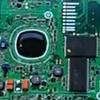# Improper Fraction to Mixed Number

propFrac fx-CG500 fx-CP500 Improper Fraction Mixed Number

3 replies to this topic

### #1sascha

sascha

Newbie

•• Members
•• 5 posts

• Calculators:
fx-CG500

Posted 07 June 2021 - 03:29 AM

What options are there to convert an improper fraction to a mixed number? Some other models seem to have a button for that to convert a result, but on the CG500 I found only the propFrac function so far.

Edited by sascha, 07 June 2021 - 03:33 AM.

### #2Hlib2

Hlib2

Casio Freak

•• Members
•• 139 posts
• Gender:Male
• Location:Ukraine
• Interests:industrial electronics,
graphing calculators

• Calculators:
fx-9860GII-2,
cfx-9850GC+,
fx-9750G+, graph_100+,
fx-9750GII, fx-991DE_X,
ti-83+_SE, ti-84+, ti-85,
ti-89_Titanium,
ti-voyage200.

Posted 09 June 2021 - 03:54 PM

I don't have a CG-500, but even in the fx-7400g+, there is a choice in the SetUp option: ab/c or d/c. Also, this option is available in all normal fx calculators.

### #3pan.gejt

pan.gejt

Casio Freak

•• Members
•• 249 posts
• Gender:Male
• Location:CZ

• Calculators:
.

Posted 11 June 2021 - 05:11 AM

Well, the propFrac command is the right tool.

propFrac(10/3) = 3+1/3 which is correct result in terms of algebra

propFrac((x^3+x^2-1)/x) = x^2+x-1/3 which is also correct result

Notice: I used brackets here since there ar no fractions template here like in calculators.

I tested first expression on ClassPad, Voyage 200 and HP-50g and all three calcs gave 3+1/3 as a result

First of all don't you need to make difference between CAS and non-CAS calculators.

The non-CAS calulators are limited by arithmetic operations (numeric operations only).

The CAS calculators can make arithmetic and algebraic operations (symbolic operations) together so such calcs follow algebraic rules.

The CAS calculators treat mixed number expressions as multiplication of two variables with omitted multiplication sign, otherwise the calc can't make symbolic calulations and symbolic manipulations- see example above.

So if you write in CAS calc 5 and 2/3, you obtain 5×2/3 = 10/3 as the result, which is expected result because calc treats the 5 and 2/3 as variables, like in similar cases: 2Pi, 5e^x,2(x+3), (x+y)(x-y).

Edited by pan.gejt, 12 June 2021 - 04:00 PM.

### #4Hlib2

Hlib2

Casio Freak

•• Members
•• 139 posts
• Gender:Male
• Location:Ukraine
• Interests:industrial electronics,
graphing calculators

• Calculators:
fx-9860GII-2,
cfx-9850GC+,
fx-9750G+, graph_100+,
fx-9750GII, fx-991DE_X,
ti-83+_SE, ti-84+, ti-85,
ti-89_Titanium,
ti-voyage200.

Posted 12 June 2021 - 01:28 PM

First of all don`t you need to make difference between CAS and non-CAS calculators

In my opinion, operations with fractions are best implemented in TI-200:
3+1/3 = 10/3
PropFrac(10/3) = 3 + 1/3
PropFrac(3+1/3) = 3 + 1/3
The only problem is that this calculator does not disable the internal CAS mode for all modes and calculations, although it always displays the results in accordance with the specified settings. This feature sometimes limits the speed of calculations in simple tasks.

### Also tagged with one or more of these keywords: propFrac, fx-CG500, fx-CP500, Improper Fraction, Mixed Number

#### 0 user(s) are reading this topic

0 members, 0 guests, 0 anonymous users